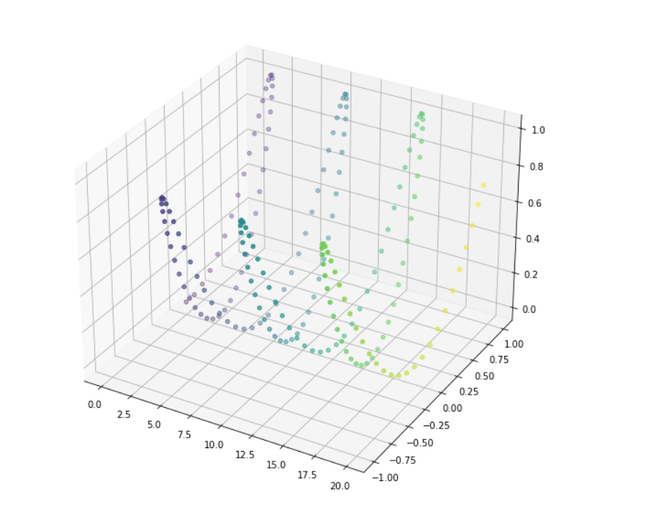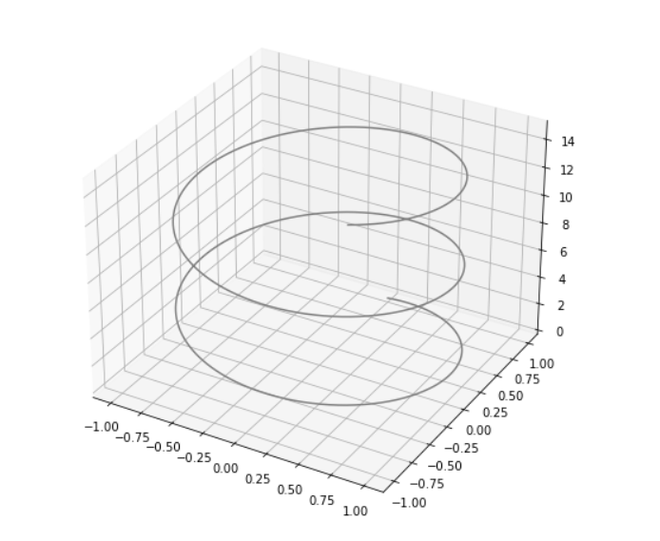# 3D Sine Wave Using Matplotlib – Python

• Last Updated : 03 Jan, 2021

Prerequisites: Mathplotlib, NumPy

In the Cartesian coordinate system, the trigonometric sine function sin(x) sin (x) generates a regular undulating curve, which passes through the origin. Its values range between −1 − 1 and 1 1 for all real values of x x.
In this article, we will plot a sin wave graph using scatter in 3-Dimension. A simple way to plot sine wave in python using matplotlib.

Approach:

• Import required libraries.
• Create an array of points.
• Plotting 3D-graph.
• Create a sin wave.
• Show Graph.

Example 1: In this example, we are plotting a 3-d graph of the sine wave signal using the scatter method.

## Python3

 `import` `numpy as np ` `import` `matplotlib.pyplot as plt ` `from` `mpl_toolkits.mplot3d ``import` `Axes3D ` ` `  `# Creating array points using numpy ` `x ``=` `np.arange(``0``, ``20``, ``0.1``) ` `y ``=` `np.sin(x) ` `z ``=` `y``*``np.sin(x) ` `c ``=` `x ``+` `y ` ` `  `#Change the Size of Graph using Figsize ` `fig ``=` `plt.figure(figsize ``=` `(``10``, ``10``)) ` ` `  `#Generating a 3D sine wave ` `ax ``=` `plt.axes(projection ``=` `'3d'``) ` ` `  `# To create a scatter graph ` `ax.scatter(x, y, z, c ``=` `c) ` ` `  `# show the graph  ` `plt.show()`

Output:Example 2: In this example, we are plotting a simple 3-D line graph of the sine wave signal.

## Python3

 `from` `mpl_toolkits ``import` `mplot3d ` `import` `numpy as np ` `import` `matplotlib.pyplot as plt ` ` `  `fig ``=` `plt.figure(figsize``=``(``8``, ``8``)) ` `ax ``=` `plt.axes(projection ``=` `'3d'``) ` ` `  `# Creating array points using numpy ` `z ``=` `np.linspace(``0``, ``15``, ``1000``) ` `x ``=` `np.sin(zline) ` `y ``=` `np.cos(zline) ` `ax.plot3D(x, y, z, ``'gray'``) ` ` `  `plt.show()`

Output:My Personal Notes arrow_drop_up
Recommended Articles
Page :